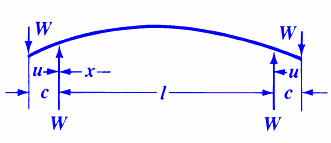Bending, Deflection and Stress Equations Calculator for
Beam Ends Overhanging Supports and a Two Equal Loads Applied at Symmetrical Locations

Beam Deflection and Stress Formula and Calculators

Structural Beam Deflection, Stress, Bending Equations and calculator for a Beam with Ends Overhanging Supports and a Two Equal Loads applied at Symmetrical Locations.Stress between loads and nearest supportStress between the two supportsStress at supports and all point along length  between supportsDeflection between supportsDeflection at the center of the beamWhere:

 E = Modulus of Elasticity psi (N/mm2) I = Moment of Inertia in4 (mm4) W = Load lbs (N) s = Stress at the cross-section being evaluated Lbs/in2 (N/mm2) y = Deflection inches (mm) x = Some distance as indicated inches (mm) u = Some distance as indicated inches (mm) c = Some distance as indicated inches (mm)= Some distance as indicated inches (mm) Z = section modulus of the cross-section of the beam = I/z in3 (mm3) z = distance from neutral axis to extreme fiber (edge) inches (mm)
• Please note letter "" (lower case "L") is different than "I" (Moment of Inertia).
• Deflections apply only to constant cross sections along entire length.

References:

• Any Machinery's Handbook published since 1931 or,
• Machinery's Handbook, 21st Edition, Page 407 or,
• Machinery's Handbook, 23st Edition, Page 263 or,
• Machinery's Handbook, 27st Edition, Page 264Membership Register | LoginHomeEngineering Book StoreEngineering ForumExcel App. DownloadsOnline Books & ManualsEngineering NewsEngineering VideosEngineering CalculatorsEngineering ToolboxGD&T Training Geometric Dimensioning TolerancingDFM DFA TrainingTraining Online EngineeringAdvertising CenterCopyright Notice